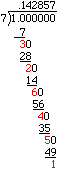Quandaries and Queries Hello my name is Leslie, I am trying to help my son with this problem and need help. In the decimal expansion of 1/17 what digit is in the 1997th place? many thanks if you can help Hi Leslie, I am going to answer the question In the decimal expansion of 1/7 what digit is in the 1997th place? and you can do 1/17. Start by dividing 7 into 1 to put 1/7 into decimal form, and look for a pattern.If you continue, the pattern "142857" repeats.You can see why if you look at the remainders, which I have in red. Once you have a remainder that appears in the list above, the pattern repeats. Here the remainder of 1 results in the repeat. Hence  1/7 = 0.142857142857142857... If you continue for 1997 places, how many times does the pattern 142857 repeat? The pattern is 6 characters in length and 1997/6 = 332 with a remainder of 5. Hence the pattern repeats 332 times and then threre are 5 more digits. They are 14257 and hence the 1997th digit in the decimal representation of 1/7 is 7. Penny Go to Math Central